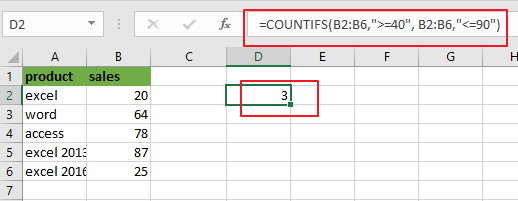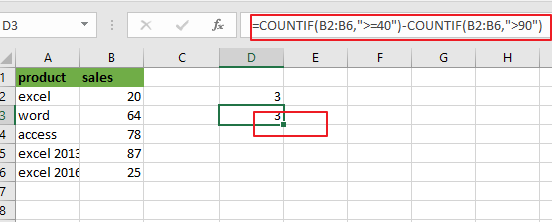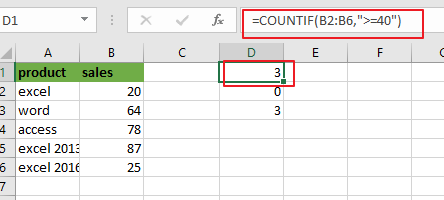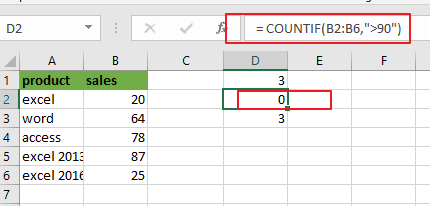# How to Count Cells between Two Numbers in Excel

This post will guide you how to count cells between two numbers using a formula in Excel 2013/2016. How do I count times between a given range cells based on a condition in Excel. Is there a easy way to count cells between two given numbers in a selected range of cells in Excel.

## Count Cells between Numbers using COUNTIFS Function

Assume that you have a list of data in range A1:B6, and you want to count cells between two numbers. In this case, and you can use the COUNTIFS function to achieve the result of counting times between two given numbers in Excel. You should know that the COUNTIFS function will count the number of cells that meet one or more criteria. And the below tutorial will help all levels of Excel users in counting cells between two given numbers in your worksheet.

You can use the following formula based on the COUNTIFS function to count cells between 40 and 90.

=COUNTIFS(B2:B6,”>=40″, B2:B6,”<=90″)From the above screenshot, and you can see that this formula will count sales number in range B2:B6 that are greater than or equal to 40 and less than or equal to 90. To count cells between two numbers, and you need to provide two criteria in COUNTIFS formula. One is for starting number and one is for the ending number.

Let’s see how this formula works:

The COUNTIFS function can be used to count cells that meet one or more criteria in the given range cells.

This formula has two criteria. It counts the cells in range of cells B2:B6 with values between 40 and 90. The operator “>=” means “greater than or equal to”, and “<=” means “less than or equal to”.

The formula will return the value “3”, which indicates to that there are three cells with values between 40 and 90 in your range of cells.

## Count Cells between Numbers using COUNTIF Function

You can also use another function called COUNTIF in an older version of Microsoft Excel to achieve the result of counting cells between two numbers. For the example above you can use the below formula based on the COUNTIF function:

=COUNTIF(B2:B6,”>=40″)-COUNTIF(B2:B6,”>90″)Let’s see that how this formula works:

The first COUNTIF function will count the number of cells in a range that are greater than or equal to “40”. And the second COUNTIF function will count the number of cells with values that are greater than “90”. And the second number is subtracted from the first result that returned by the first COUNTIF function. Then you should get the number of cells that contain values between 40 and 90.

The First COUNTIF function:

=COUNTIF(B2:B6,”>=40″)The Second COUNTIF function:

= COUNTIF(B2:B6,”>90″)### Related Functions

• Excel COUNTIF function
The Excel COUNTIF function will count the number of cells in a range that meet a given criteria. This function can be used to count the different kinds of cells with number, date, text values, blank, non-blanks, or containing specific characters.etc.= COUNTIF (range, criteria)…
• Excel COUNTIFS function
The Excel COUNTIFS function returns the count of cells in a range that meet one or more criteria. The syntax of the COUNTIFS function is as below:= COUNTIFS(criteria_range1, criteria1, [criteria_range2, criteria2]…)…